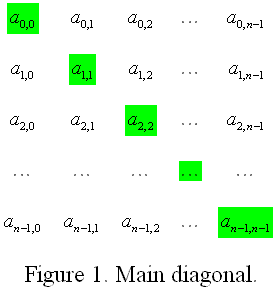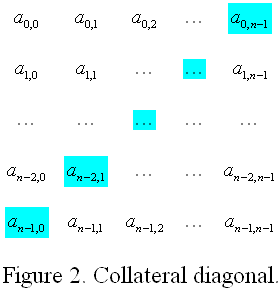In this section i will share several problem sets programming that I’ve ever done, you will see the problem and the solution in C language programming on the bottom. In another occasion i will share another problem sets but NOT include the solution. I hope my post today can bring some references. Thanks have nice day.

Problem bellow I was found in my test selection for joining some occasion, I think this is good experience can done this test and i share with the problem. Begin with Diagonal Disproportion Problem and Solution

## Diagonal Disproportion

Time limit: 2000 ms

Memory limit: 65536 KB

### Description

You are to calculate the diagonal disproportion of a square matrix. The diagonal disproportion of a square matrix is the sum of the elements of its main diagonal minus the sum of the elements of its collateral diagonal. The main and collateral diagonals of a square matrix are shown in figures 1 and 2 respectively.The elements of the main diagonal are shown in green in figure 1, and the elements of the collateral diagonal are shown in cyan in figure 2.

Given a matrix, return its diagonal disproportion. The j’th character of the i’th element of matrix should be treated as the element in the i’th row and j’th column of the matrix.

### Input Format

First line consists of one integer N (1 <= N <= 50), the size of the matrix. The next N lines consist of N 1-digit numbers

### Output Format

One integer in one line, that is the diagonal disproportion of that matrix.

```3
190
828
373```

`1`

### Note

The sum of the elements of the main diagonal is 1+2+3 = 6. The sum of the elements of the collateral diagonal is 0+2+3 = 5. So, the answer is 6-5 = 1.

```4
9000
0120
0000
9000```

`-1`

```10
7748297018
8395414567
7006199788
5446757413
2972498628
0508396790
9986085827
2386063041
5687189519
7729785238```

`-24`

# Solution

```#include <stdio.h>
#include <stdlib.h>

int main(){
int N;
scanf("%d",&N);
if(N>=1 && N <=50){
char temp[N];
char x;
int i,a, result1 = 0, result2 = 0;
for(i = 0 ; i < N;i++){
fflush(stdin);
scanf("%s",&temp);
x = temp[i];
a = x - '0';
result1 += a;
x = temp[N-(i+1)];
a = x - '0';
result2 += a;
}
printf("%d\n",result1-result2);
}
return 0;
}
```
Programming Problem Test #Diagonal Disproportion